# Cross Multiply

Cross Multiply

Do you know how to solve equations with fractions that too by multiplication?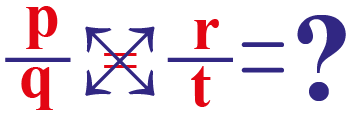The answer is using cross multiply, an easy and simple method to get the answers in seconds.

In this mini-lesson, we will explore the world of cross multiply by finding the answers to the questions like what is meant by cross multiplication, how to do a cross multiply, and how to do a cross multiply with variables with the help of interactive questions.

## Lesson Plan

 1 What Do You Mean By Cross Multiply? 2 Important Notes on Cross Multiply 3 Solved Examples on Cross Multiply 4 Interactive Questions on Cross Multiply 5 Challenging Question on Cross Multiply

## What Is Meant By Cross Multiplication?

### Cross-Multiply Definition

When two fractions $$\dfrac{p}{q}$$ and $$\dfrac{r}{t}$$ are multiply by each other is termed as cross-multiplication method.

In the cross-multiplication method, the numerator of the first fraction value is multiplied by the denominator of the second fraction.

It can be termed as a cross-multiplication method or a cross multiply.

### Cross-Multiply Formula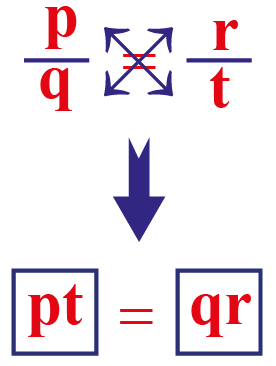## How To Cross Multiply?

Let us learn how to do cross multiply. Consider the example, $$\dfrac{4}{6}=\dfrac{2}{3}$$

Step 1

Multiply the numerator of the right-hand side fraction value with the denominator of the left-hand side fraction value.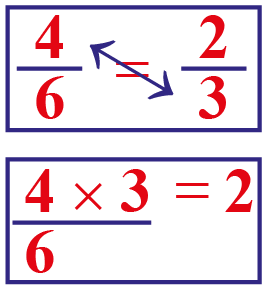Step 2

Multiply the denominator of the right-hand side fraction value with the numerator of the left-hand side fraction value.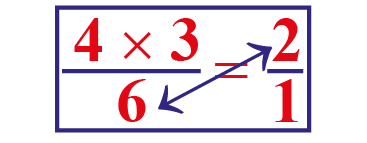Step 3

LHS is equal to RHS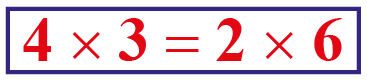$$12=12$$

## How To Cross Multiply With A Variable?

Let us learn how to do cross multiply with a variable. Consider the example, $$\dfrac{x}{6}=\dfrac{6}{x}$$

We will be using the general cross multiply formula,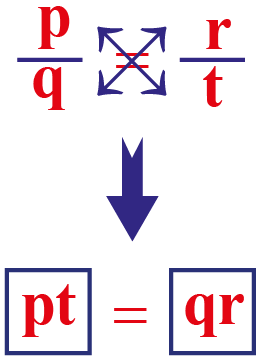$$\dfrac{x}{6}=\dfrac{6}{x}$$

Multiply the numerator of the right-hand side fraction value with the denominator of the left-hand side fraction value and vice-vera.

$$x^{2}=6\times6$$

$$x^{2}=36$$

$$x=\pm6$$

More Important Topics
Numbers
Algebra
Geometry
Measurement
Money
Data
Trigonometry
Calculus